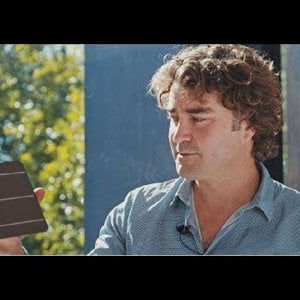1. ### Solarcells winter production

I live at 60 degrees north. On december 22nd the sun is only 8 degrees above the horizon at noon. Here lots of people say they would go off grid if they just could have a reliable electricity production during the winter. All the sellers of solar cells talks about maximizing the cells production...
2. ### Second reflection angle of incidences in 3D

Given Theta1(angle of incidence) and alpha1(azimuth angle). how do we obtain the second reflection angle theta3 and alpha3? Assuming the surface to be a mirror reflection(theta1 = theta2). Need an equation when varied the incident angles we would obtain the second reflection angles or a method...
3. ### Perovskite solar cell J-V scan

Hi all, I am working on perovskite solar cell related studies. J-V curve (current density-voltage curve) is one of the important measurements to determine the performance of the device. In practice, we perform the reverse scan first then then followed by the forward scan. There is usually a...
4.### The Path to Perovskite on Silicon PV | Prof. Henry Snaith

In this interview, Prof. Henry Snaith (University of Oxford) discusses the present status and future prospects of perovskite PV. Article: https://scivpro.com/manuscript/10_32386_scivpro_000004/
5. ### B Solar cells - how do they repeat the process?

Hey everyone, I have to do this presentation about solar cells and how they work. I've been doing a lot of research, and now I know a lot about how they work. However there is just one thing, that I can't understand. So in a solar cell there is an N-type and P-Type. The N-type has too many...